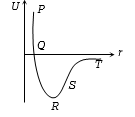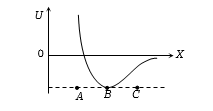K is the force constant of a spring. The work done in increasing its extension from ${l}_{1}$ to ${l}_{2}$ will be

(a) $K\left({l}_{2}-{l}_{1}\right)$                             (b) $\frac{K}{2}\left({l}_{2}+{l}_{1}\right)$

(c) $K\left({l}_{2}^{2}-{l}_{1}^{2}\right)$                            (d) $\frac{K}{2}\left({l}_{2}^{2}-{l}_{1}^{2}\right)$

Concept Questions :-

Elastic potential energy
High Yielding Test Series + Question Bank - NEET 2020

Difficulty Level:

The points of maximum and minimum attraction in the curve between potential energy (U) and distance (r) of a diatomic molecules are respectively -(a) Sand R

(b) T and S

(c) R and S

(d) S and T

Concept Questions :-

Potential energy: Relation with force
High Yielding Test Series + Question Bank - NEET 2020

Difficulty Level:

The potential energy U between two molecules as a function of the distance X between them has been shown in the figure. The two molecules are -1. Attracted when x lies between A and B and are repelled when X lies between B and C

2. Attracted when x lies between B and C and are repelled when X lies between A and B

3. Attracted when they reach B

4. Repelled when they reach B

Concept Questions :-

Potential energy: Relation with force
High Yielding Test Series + Question Bank - NEET 2020

Difficulty Level:

Water falls from a height of 60m at the rate of 15kg/s to operate a turbine. The losses due to frictional forces are 10% of energy. How much power is generated by the turbine? (g=10 m/${s}^{2}$)

1. 8.1 kW                                        2. 10.2 kW

3. 12.3 kW                                      4. 7.0 kW

Concept Questions :-

Power
High Yielding Test Series + Question Bank - NEET 2020

Difficulty Level:

A block of mass M is attached to the lower end of a vertical spring. The spring is hung from a ceiling and has force constant value k. The mass is released from rest with the spring initially unstretched. The maximum extension produced in the length of the spring will be

(a) Mg/k

(b) 2Mg/k

(c) 4 Mg/k

(d) Mg/2k

Concept Questions :-

Work-Energy theorem
High Yielding Test Series + Question Bank - NEET 2020

Difficulty Level:

A body of mass 1 kg is thrown upwards with a velocity  It momentarily comes to rest after attaining a height of 18 m. How much energy is lost due to air friction?

(a) 20 J                               (b) 30 J

(c) 40 J                                (d) 10 J

Concept Questions :-

Work-Energy theorem
High Yielding Test Series + Question Bank - NEET 2020

Difficulty Level:

A particle of mass M starting from rest undergoes uniform acceleration. If the speed acquired in time T is v, the power delivered to the particle is

(a) $\frac{{\mathrm{Mv}}^{2}}{\mathrm{T}}$                                  (b) $\frac{1}{2}\frac{{\mathrm{Mv}}^{2}}{{\mathrm{T}}^{2}}$

(c) $\frac{{\mathrm{Mv}}^{2}}{{\mathrm{T}}^{2}}$                                   (d) $\frac{1}{2}\frac{{\mathrm{Mv}}^{2}}{\mathrm{T}}$

Concept Questions :-

Power
High Yielding Test Series + Question Bank - NEET 2020

Difficulty Level:

An engine pumps water through a hosepipe. Water passes through the pipe and leaves it with a velocity of 2 $m{s}^{-1}.$The mass per unit length of water in the pipe is 100 $kg{m}^{-1}.$What is the power of the engine?

(a) 400W                                        (b) 200W

(c) 100W                                        (d) 800W

Concept Questions :-

Power
High Yielding Test Series + Question Bank - NEET 2020

Difficulty Level:

A ball moving with velocity  collides head on with another stationery ball of double the mass. If the coefficient of restitution is 0.5, then their velocities (in $m{s}^{-1}$) after collision will be

(a)0,1                                                (b)1,1

(c)1,0.5                                             (d)0,2

Concept Questions :-

Application of laws
High Yielding Test Series + Question Bank - NEET 2020

Difficulty Level:

The potential energy of a system increases if work is done

(a) by the system against a conservative force

(b) by the system against a nonconservative force

(c) upon the system by a conservative force

(d) upon the system by a nonconservative force

Concept Questions :-

Potential energy: Relation with force